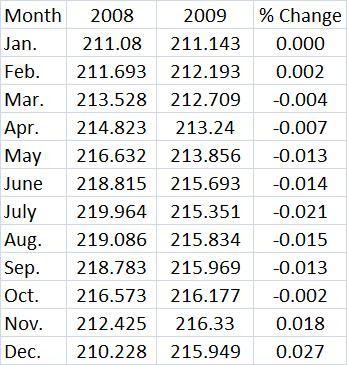## Wednesday, February 24, 2010

### What Was The Rate Of Inflation Last Year? Was It Positive Or Negative?

First, a note to my students. I added some links and informaton to the last post on religion and economics.

This may not be as simple a question as you might guess. You can get data on this from the Bureau of Labor Statistics at Consumer Price Index. The official rate is 2.7%. Why?

In December of 2008, the CPI = 210.228. In December of 2009, the CPI = 215.949. The latter number is 2.7% higher than the first number. But, what if we took the average CPI of all 12 months in each year and then found the percentage change?

The average CPI for all 12 months of 2008 was 215.303. The average for 2009 was 214.537. Notice that the 2nd number is lower. How much lower? 214.537/214.537 = 0.99644. That means that prices were only 99.644% as high in 2009 as they were in 2008. The percentage drop was about 0.356% since 1 - 0.99644 = 0.00356.

The table below shows the CPI in each month for each year and then the % change month-to-month going forward a year.When you look at the BLS web site linked above, you will see in the last column a -0.4 or a -0.4% (the site does not put any % signs in). That is just rounded up slightly from the actual -0.356%. The last year there was a fall in the CPI, whether by the December-to-December method or the monthly average method was 1955 (it was only the monthly average that was negative).

Usually, the two methods are within 1 percentage point of each other (58 out of 96 years). But in each of the last two years, they differed by more than 3 percentage points (in 2008, the Dec-Dec rate was 0.1% while it was 3.8% using monthly averages). The last time there were two straight years like that was from 1946-48. So we must be having some pretty big month-to-month fluctuations. The graph below shows the absolute difference between the two methods for each year since 1914. Notice that the differences became larger in the last few years.